# 978-0073382395 Chapter 13 Questions and Problems 18-28

Document Type
Homework Help
Book Title
Fundamentals of Corporate Finance Standard Edition 9th Edition
Authors
Stephen Ross
B-242 SOLUTIONS
b. We need to find the portfolio weights that result in a portfolio with a of 0.95. We know the of
the risk-free asset is zero. We also know the weight of the risk-free asset is one minus the weight
of the stock since the portfolio weights must sum to one, or 100 percent. So:
p = 0.95 = wS(1.35) + (1 – wS)(0)
0.95 = 1.35wS + 0 – 0wS
w
S = 0.95/1.35
w
S = .7037
And, the weight of the risk-free asset is:
w
Rf = 1 – .7037 = .2963
c. We need to find the portfolio weights that result in a portfolio with an expected return of 8 percent.
We also know the weight of the risk-free asset is one minus the weight of the stock since the
portfolio weights must sum to one, or 100 percent. So:
E(Rp) = .08 = .16wS + .048(1 – wS)
.08 = .16wS + .048 – .048wS
.032 = .112wS
w
S = .2857
So, the
of the portfolio will be:
p = .2857(1.35) + (1 – .2857)(0) = 0.386
d. Solving for the of the portfolio as we did in part a, we find:
p = 2.70 = wS(1.35) + (1 – wS)(0)
w
S = 2.70/1.35 = 2
w
Rf = 1 – 2 = –1
The portfolio is invested 200% in the stock and –100% in the risk-free asset. This represents
borrowing at the risk-free rate to buy more of the stock.
18. First, we need to find the of the portfolio. The of the risk-free asset is zero, and the weight of the
risk-free asset is one minus the weight of the stock, the of the portfolio is:
ß
p = wW(1.25) + (1 – wW)(0) = 1.25wW
So, to find the of the portfolio for any weight of the stock, we simply multiply the weight of the stock
times its .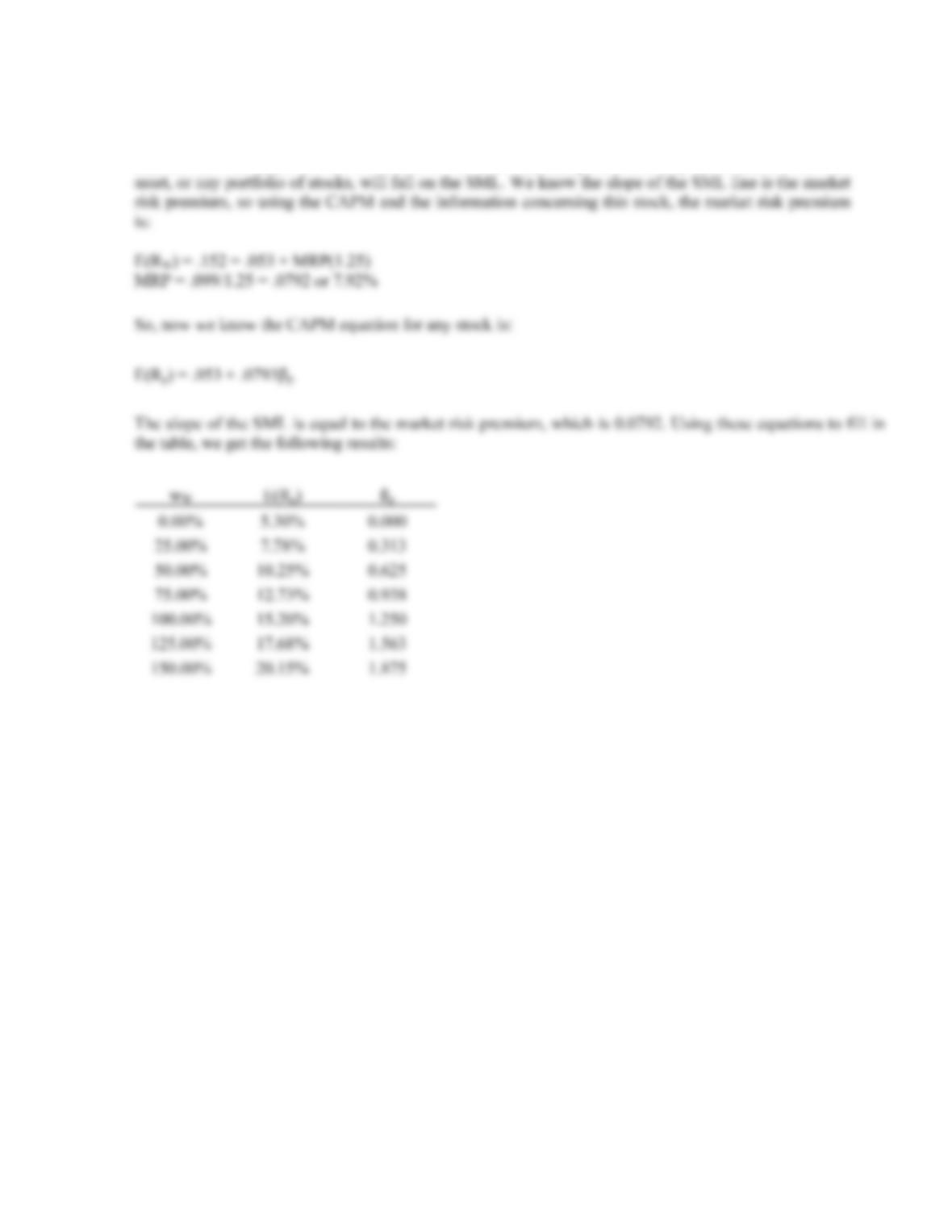CHAPTER 13 B-243
Even though we are solving for the and expected return of a portfolio of one stock and the risk-free
asset for different portfolio weights, we are really solving for the SML. Any combination of this stock,
and the risk-free asset will fall on the SML. For that matter, a portfolio of any stock and the risk-free
19. There are two ways to correctly answer this question. We will work through both. First, we can use the
CAPM. Substituting in the value we are given for each stock, we find:
E(RY) = .08 + .075(1.30) = .1775 or 17.75%
It is given in the problem that the expected return of Stock Y is 18.5 percent, but according to the
CAPM, the return of the stock based on its level of risk, the expected return should be 17.75 percent.
This means the stock return is too high, given its level of risk. Stock Y plots above the SML and is
undervalued. In other words, its price must increase to reduce the expected return to 17.75 percent. For
Stock Z, we find:
E(RZ) = .08 + .075(0.70) = .1325 or 13.25%
The return given for Stock Z is 12.1 percent, but according to the CAPM the expected return of the
stock should be 13.25 percent based on its level of risk. Stock Z plots below the SML and is overvalued.
In other words, its price must decrease to increase the expected return to 13.25 percent.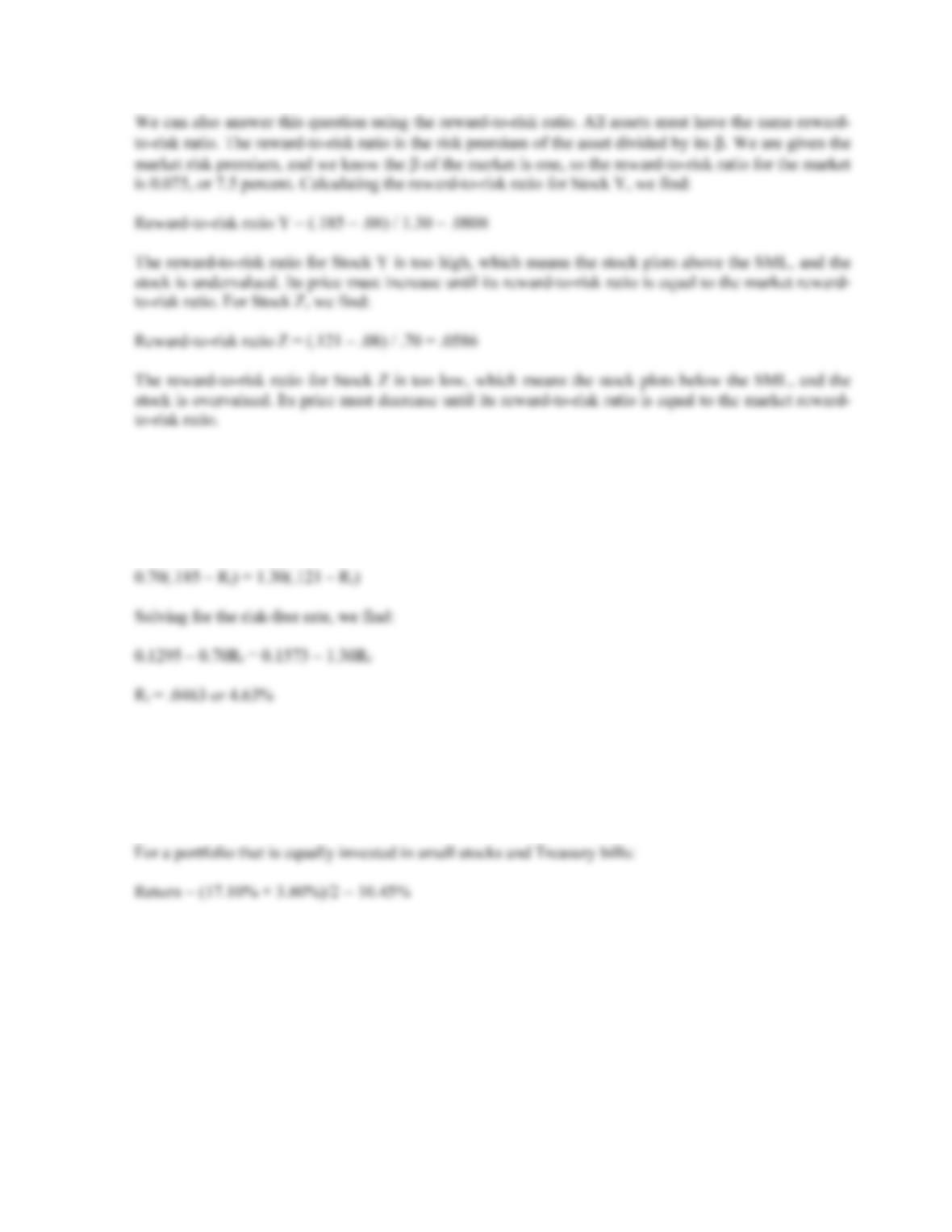B-244 SOLUTIONS
20. We need to set the reward-to-risk ratios of the two assets equal to each other, which is:
(.185 Rf)/1.30 = (.121 – Rf)/0.70
We can cross multiply to get:
Intermediate
21. For a portfolio that is equally invested in large-company stocks and long-term bonds:
Return = (12.30% + 5.80%)/2 = 9.05%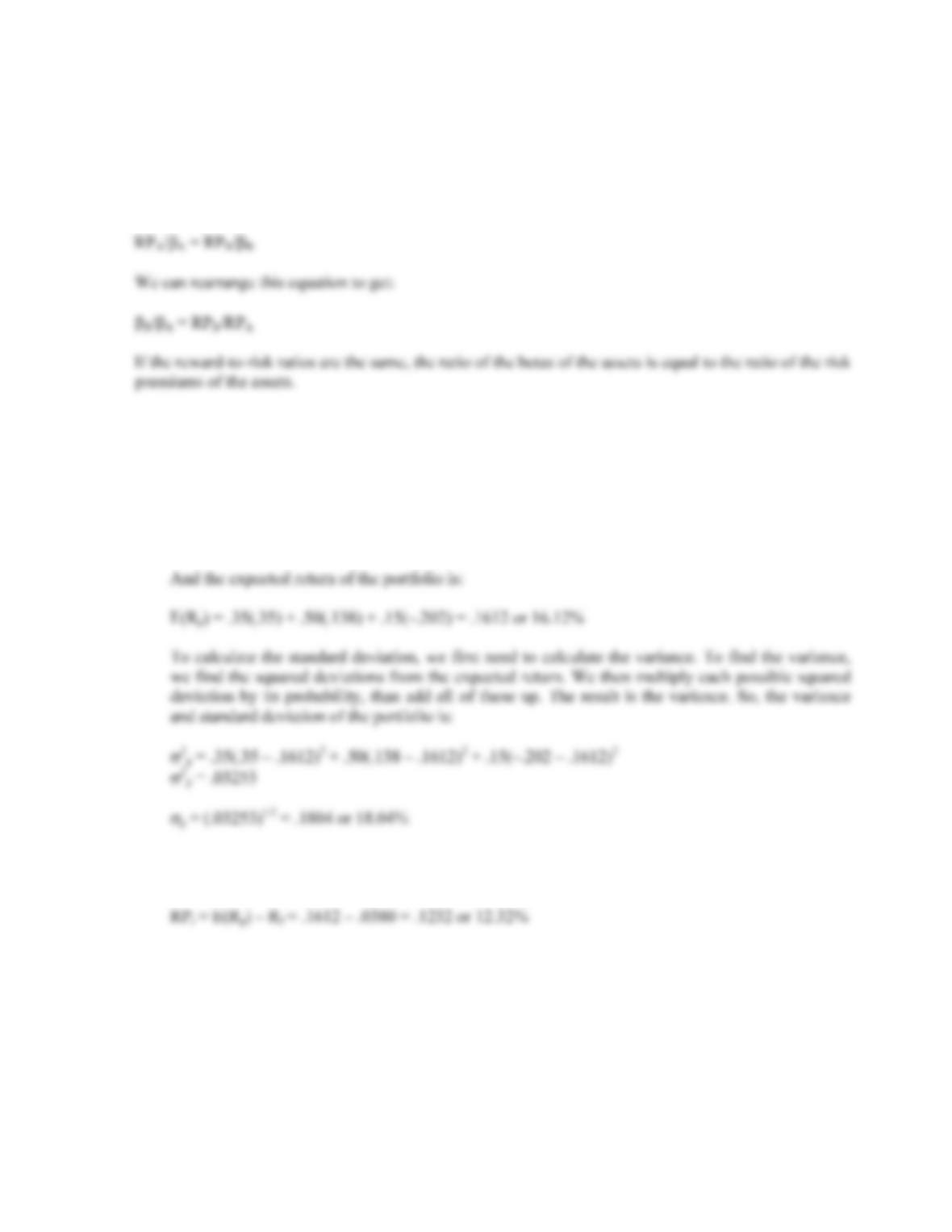CHAPTER 13 B-245
22. We know that the reward-to-risk ratios for all assets must be equal. This can be expressed as:
[E(RA) – Rf]/A = [E(RB) – Rf]/ßB
The numerator of each equation is the risk premium of the asset, so:
23. a. We need to find the return of the portfolio in each state of the economy. To do this, we will
multiply the return of each asset by its portfolio weight and then sum the products to get the
portfolio return in each state of the economy. Doing so, we get:
Boom: E(Rp) = .4(.24) + .4(.36) + .2(.55) = .3500 or 35.00%
Normal: E(Rp) = .4(.17) + .4(.13) + .2(.09) = .1380 or 13.80%
Bust: E(Rp) = .4(.00) + .4(–.28) + .2(–.45) = –.2020 or –20.20%
b. The risk premium is the return of a risky asset, minus the risk-free rate. T-bills are often used as
the risk-free rate, so: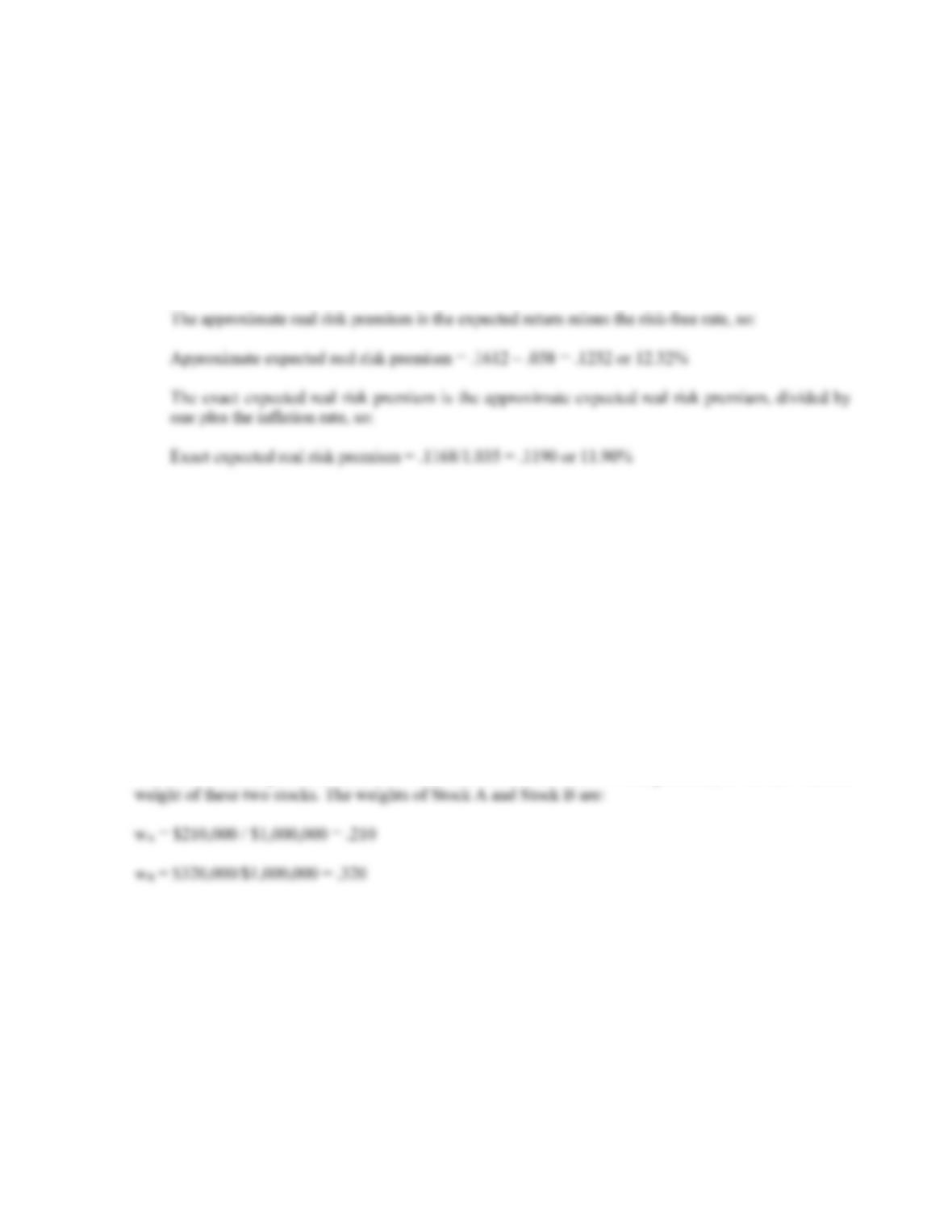B-246 SOLUTIONS
c. The approximate expected real return is the expected nominal return minus the inflation rate, so:
Approximate expected real return = .1612 – .035 = .1262 or 12.62%
To find the exact real return, we will use the Fisher equation. Doing so, we get:
1 + E(Ri) = (1 + h)[1 + e(ri)]
1.1612 = (1.0350)[1 + e(ri)]
e(ri) = (1.1612/1.035) – 1 = .1219 or 12.19%
24. Since the portfolio is as risky as the market, the of the portfolio must be equal to one. We also know the
of the risk-free asset is zero. We can use the equation for the of a portfolio to find the weight of the third
stock. Doing so, we find:
p = 1.0 = wA(.85) + wB(1.20) + wC(1.35) + wRf(0)
Solving for the weight of Stock C, we find:
w
C = .324074
So, the dollar investment in Stock C must be:
Invest in Stock C = .324074(\$1,000,000) = \$324,074.07
We know the total portfolio value and the investment of two stocks in the portfolio, so we can find the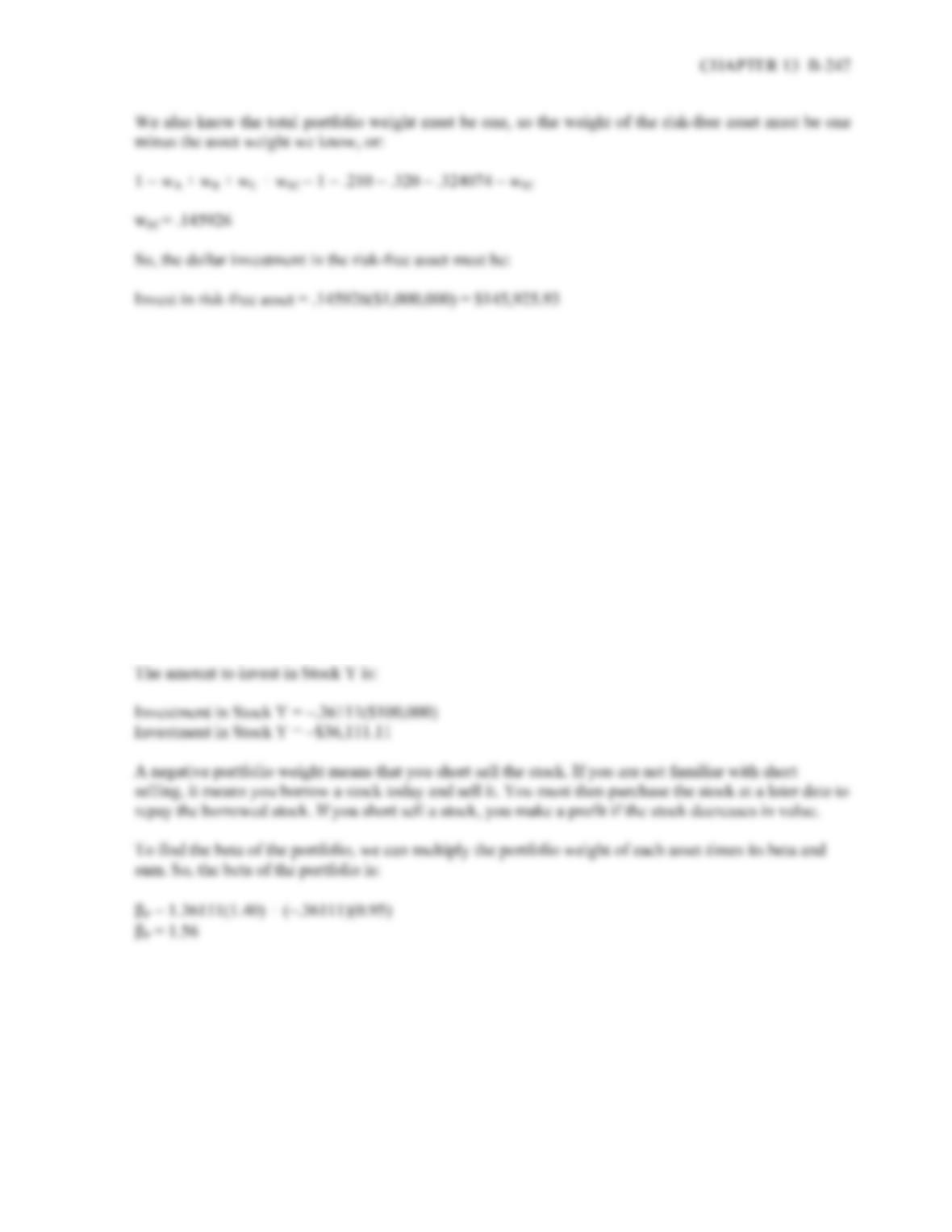Challenge
25. We are given the expected return of the assets in the portfolio. We also know the sum of the weights of
each asset must be equal to one. Using this relationship, we can express the expected return of the
portfolio as:
E(Rp) = .185 = wX(.172) + wY(.136)
.185 = wX(.172) + (1 – wX)(.136)
.185 = .172wX + .136 – .136wX
.049 = .036wX
w
X = 1.36111
And the weight of Stock Y is:
w
Y = 1 – 1.36111
w
Y = –.36111
.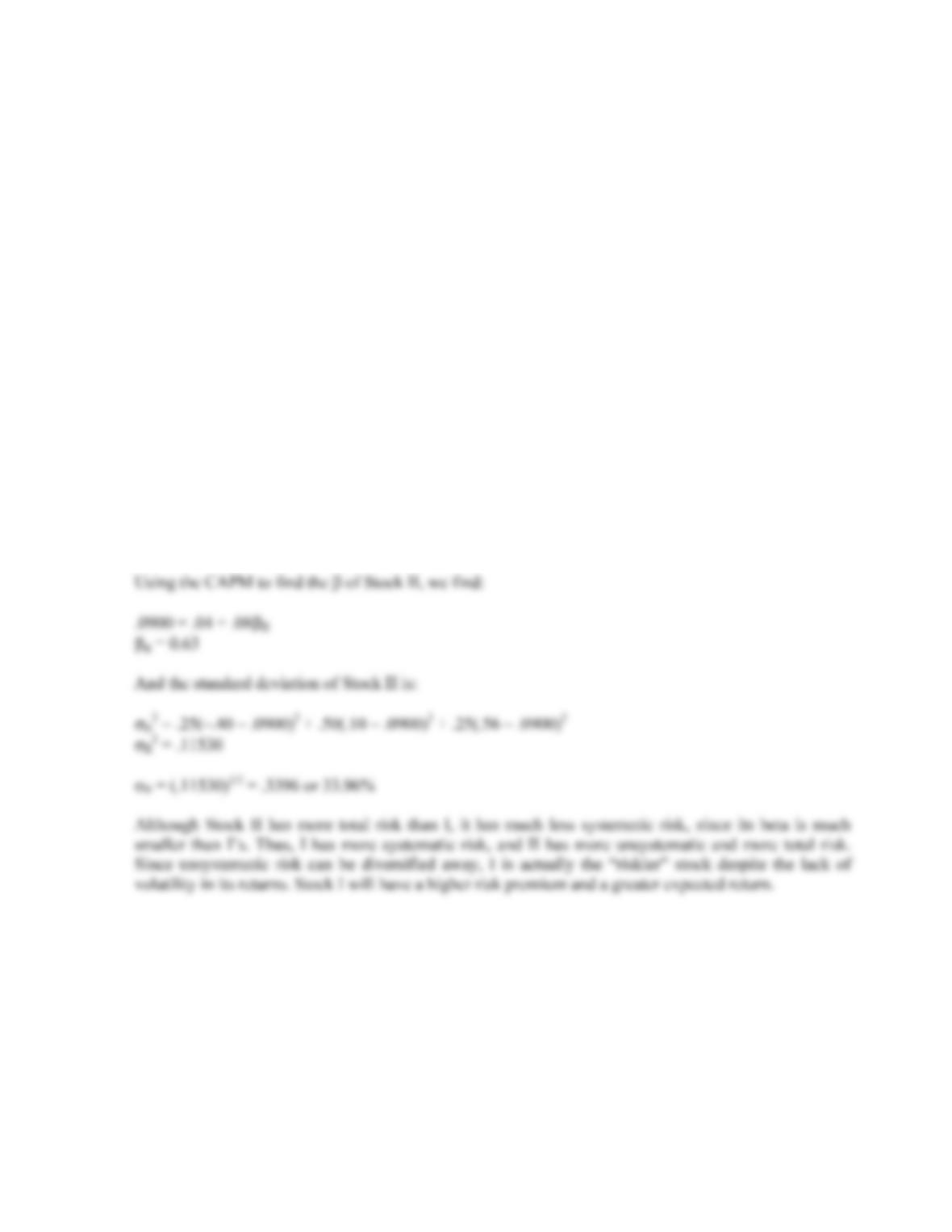B-248 SOLUTIONS
26. The amount of systematic risk is measured by the of an asset. Since we know the market risk premium
and the risk-free rate, if we know the expected return of the asset we can use the CAPM to solve for the
of the asset. The expected return of Stock I is:
E(RI) = .25(.11) + .50(.29) + .25(.13) = .2050 or 20.50%
Using the CAPM to find the of Stock I, we find:
.2050 = .04 + .08I
I = 2.06
The total risk of the asset is measured by its standard deviation, so we need to calculate the standard
deviation of Stock I. Beginning with the calculation of the stock’s variance, we find:
I2 = .25(.11 – .2050)2 + .50(.29 – .2050)2 + .25(.13 – .2050)2
I2 = .00728
I = (.00728)1/2 = .0853 or 8.53%
Using the same procedure for Stock II, we find the expected return to be:
E(RII) = .25(–.40) + .50(.10) + .25(.56) = .0900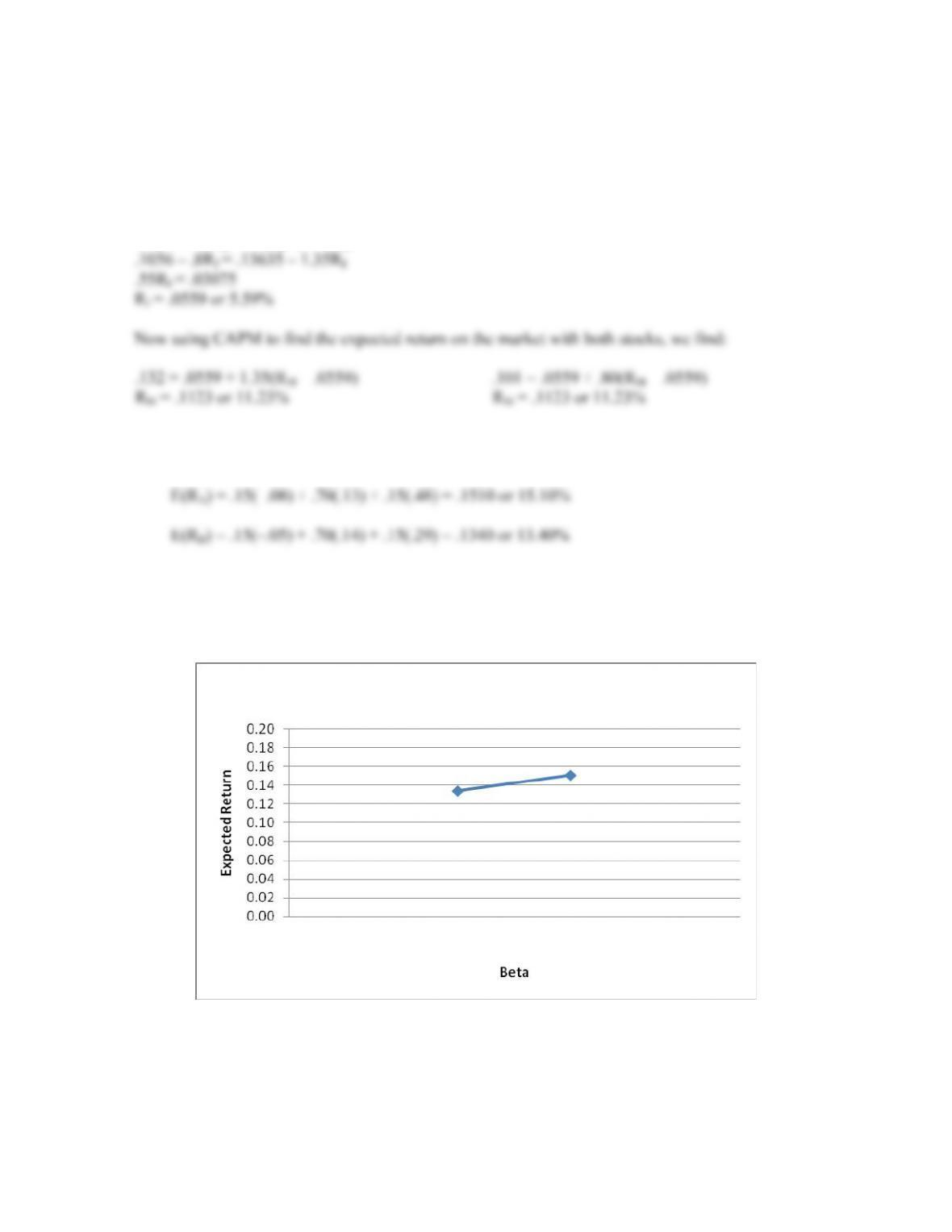CHAPTER 13 B-249
27. Here we have the expected return and beta for two assets. We can express the returns of the two assets
using CAPM. If the CAPM is true, then the security market line holds as well, which means all assets
have the same risk premium. Setting the risk premiums of the assets equal to each other and solving for
the risk-free rate, we find:
(.132 Rf)/1.35 = (.101 – Rf)/.80
.80(.132 – Rf) = 1.35(.101 – Rf)
28. a. The expected return of an asset is the sum of the probability of each return occurring times the
probability of that return occurring. So, the expected return of each stock is:
b. We can use the expected returns we calculated to find the slope of the Security Market Line. We
know that the beta of Stock A is .25 greater than the beta of Stock B. Therefore, as beta increases
by .25, the expected return on a security increases by .017 (= .1510 – .1340). The slope of the
security market line (SML) equals:
SlopeSML = Rise / Run
SlopeSML = Increase in expected return / Increase in beta
SlopeSML = (.1510 – .1340) / .25
SlopeSML = .0680 or 6.80%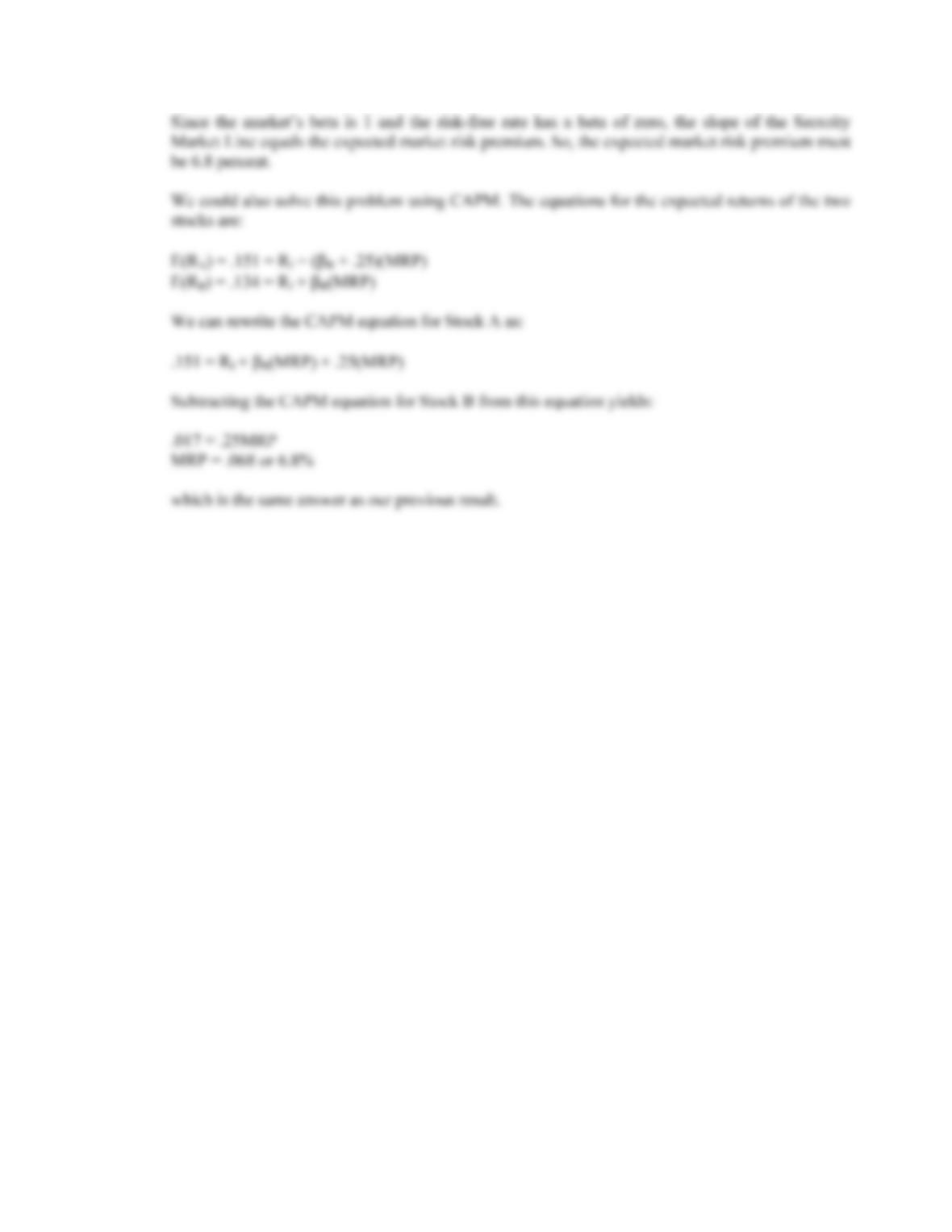B-250 SOLUTIONS

## Trusted by Thousands ofStudents

Here are what students say about us.